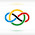### 2016 India IMO Training Camp #3

Let $a,b,c,d$ be real numbers satisfying $|a|,|b|,|c|,|d|>1$ and $abc+abd+acd+bcd+a+b+c+d=0$. Prove that $\frac {1} {a-1}+\frac {1} {b-1}+ \frac {1} {c-1}+ \frac {1} {d-1} >0$

The given equality can be manipulated as $(a + 1)(b + 1)(c + 1)(d + 1) = (a - 1)(b - 1)(c - 1)(d - 1).$Because $a, b, c, d \neq[-1, 1]$, we know that $\tfrac{a + 1}{a - 1}, \tfrac{b + 1}{b - 1}, \tfrac{c + 1}{c - 1}, \tfrac{d + 1}{d - 1}>0$ so we use AM-GM inequality to obtain $\frac{a + 1}{a - 1} + \frac{b + 1}{b - 1} + \frac{c + 1}{c - 1} + \frac{d + 1}{d - 1} \ge 4 \implies \frac{1}{a - 1} + \frac{1}{b - 1} + \frac{1}{c - 1} + \frac{1}{d - 1} \ge 0.$We have equality only when $\tfrac{a + 1}{a - 1} = \tfrac{b + 1}{b - 1} = \tfrac{c + 1}{c - 1} = \tfrac{d + 1}{d - 1} \iff a = b = c = d$, which is impossible due to the given inequality.

So, we must have $$\frac{1}{a-1}+\frac{1}{b-1}+\frac{1}{c-1}+\frac{1}{d-1}>0,$$as desired. $\square$

1.orz

2.Thanks!

3.this problem seems familiar
nice solution

4.@Unknown

I know, right? I felt the same way.

Thanks :)

5.Anonymous7/04/2022

nice job